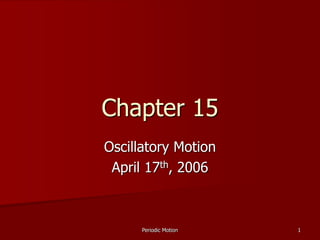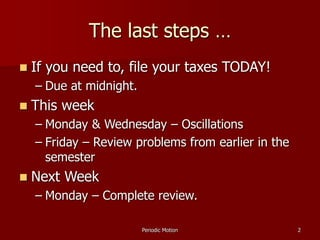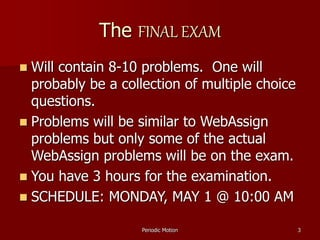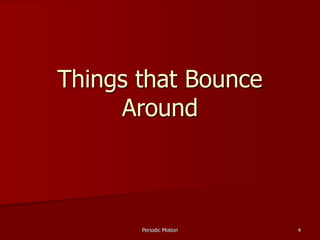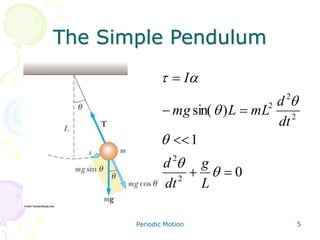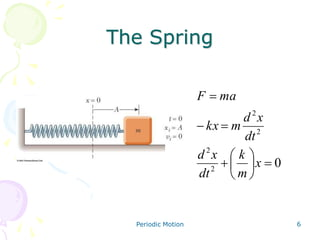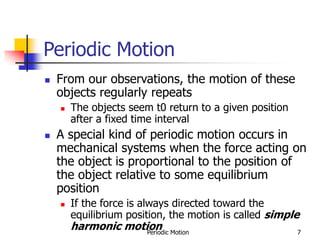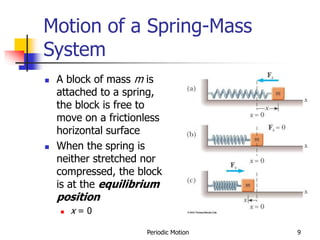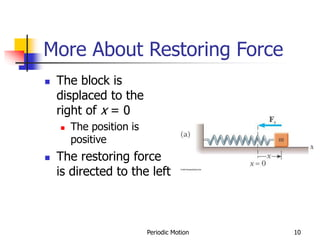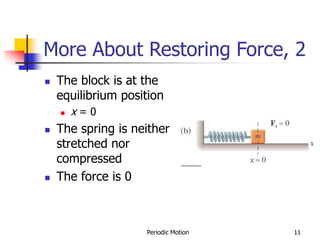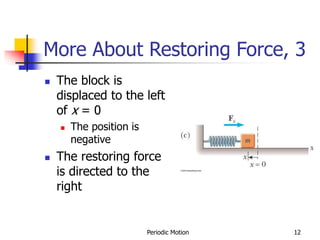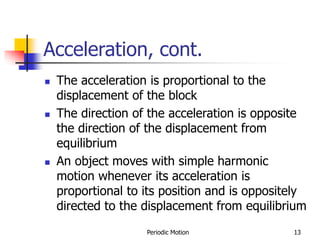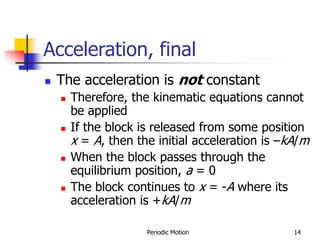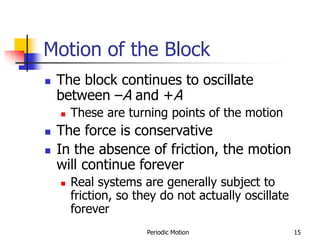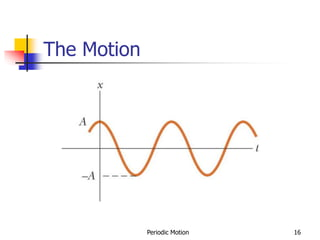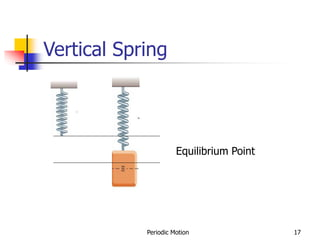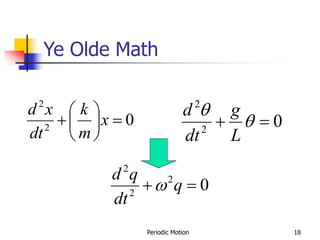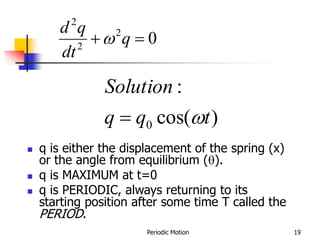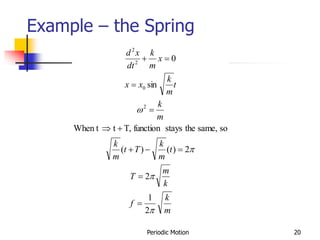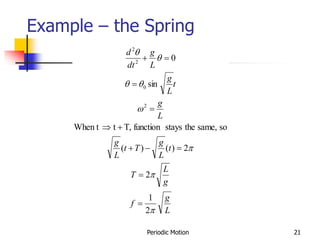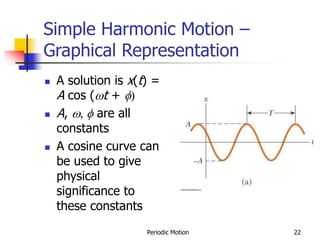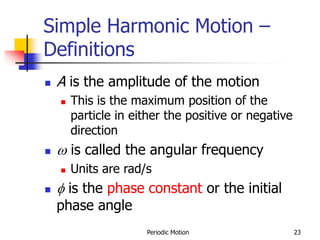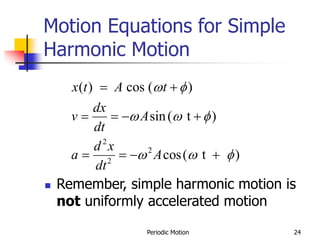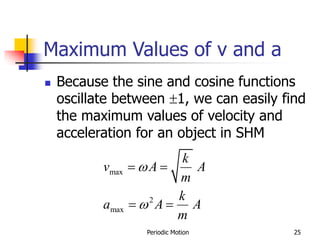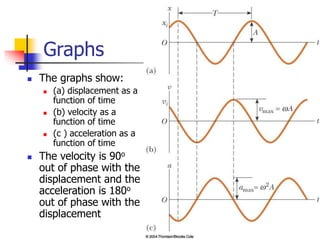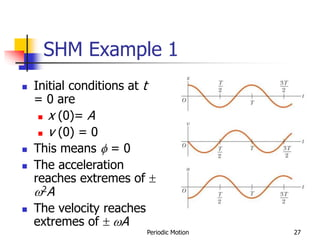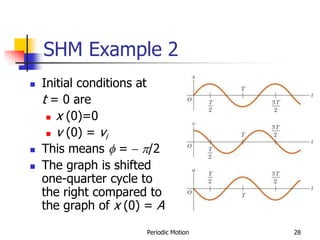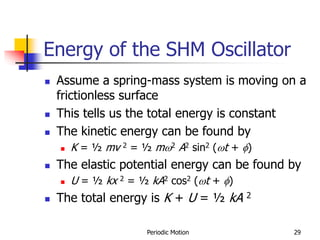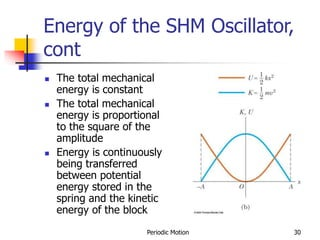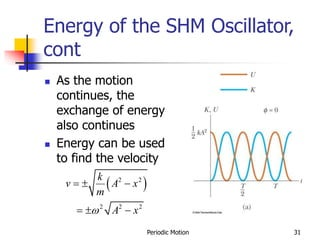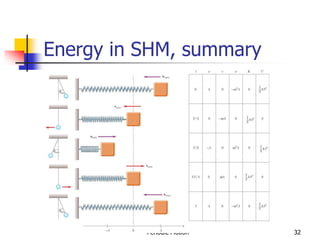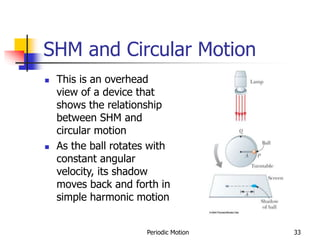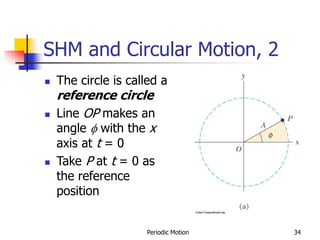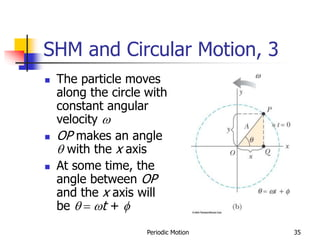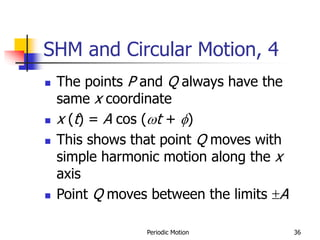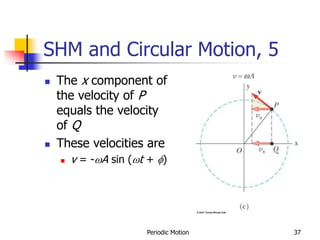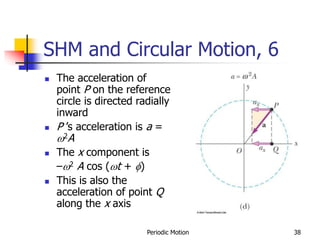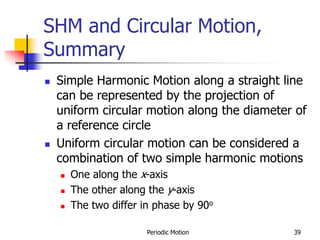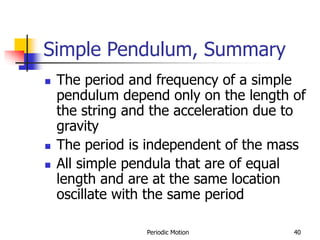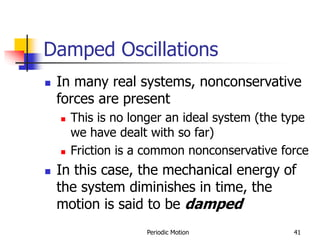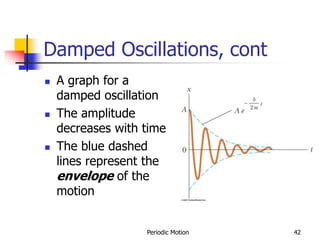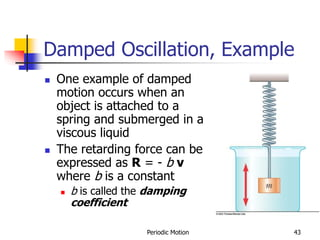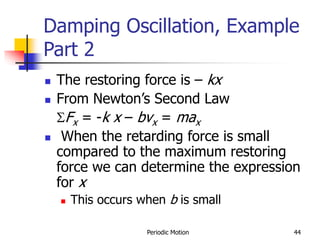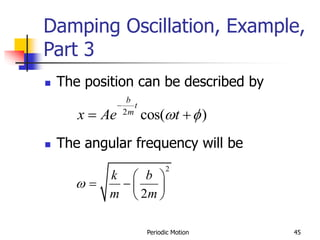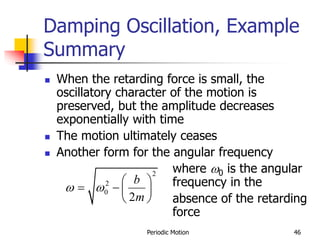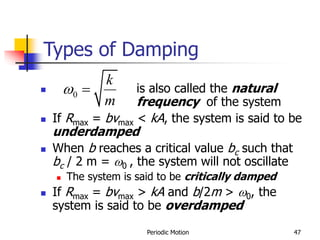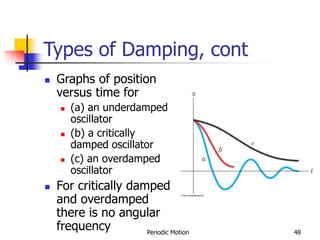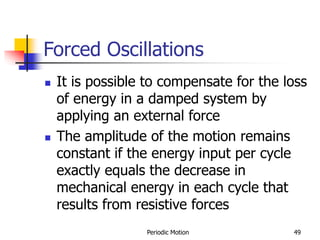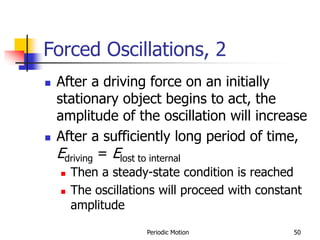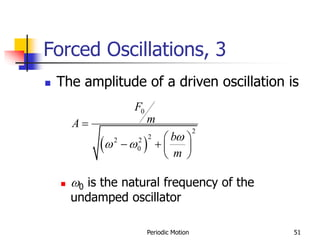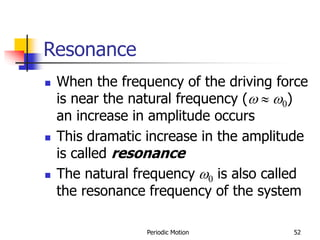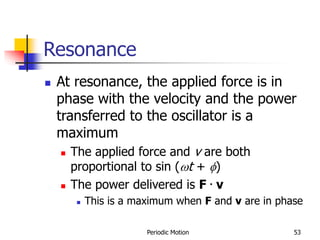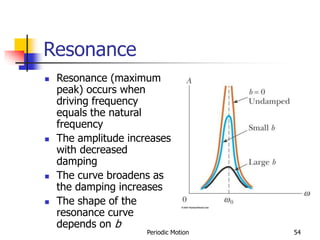1 of 55

### Periodic Motion.ppt

1. Periodic Motion 1 Chapter 15 Oscillatory Motion April 17th, 2006
2. Periodic Motion 2 The last steps …  If you need to, file your taxes TODAY! – Due at midnight.  This week – Monday & Wednesday – Oscillations – Friday – Review problems from earlier in the semester  Next Week – Monday – Complete review.
3. Periodic Motion 3 The FINAL EXAM  Will contain 8-10 problems. One will probably be a collection of multiple choice questions.  Problems will be similar to WebAssign problems but only some of the actual WebAssign problems will be on the exam.  You have 3 hours for the examination.  SCHEDULE: MONDAY, MAY 1 @ 10:00 AM
4. Periodic Motion 4 Things that Bounce Around
5. Periodic Motion 5 The Simple Pendulum 0 1 ) sin( 2 2 2 2 2              L g dt d dt d mL L mg I
6. Periodic Motion 6 The Spring 0 2 2 2 2            x m k dt x d dt x d m kx ma F
7. Periodic Motion 7 Periodic Motion  From our observations, the motion of these objects regularly repeats  The objects seem t0 return to a given position after a fixed time interval  A special kind of periodic motion occurs in mechanical systems when the force acting on the object is proportional to the position of the object relative to some equilibrium position  If the force is always directed toward the equilibrium position, the motion is called simple harmonic motion
8. Periodic Motion 8 The Spring … for a moment  Let’s consider its motion at each point.  What is it doing?  Position  Velocity  Acceleration
9. Periodic Motion 9 Motion of a Spring-Mass System  A block of mass m is attached to a spring, the block is free to move on a frictionless horizontal surface  When the spring is neither stretched nor compressed, the block is at the equilibrium position  x = 0
10. Periodic Motion 10 More About Restoring Force  The block is displaced to the right of x = 0  The position is positive  The restoring force is directed to the left
11. Periodic Motion 11 More About Restoring Force, 2  The block is at the equilibrium position  x = 0  The spring is neither stretched nor compressed  The force is 0
12. Periodic Motion 12 More About Restoring Force, 3  The block is displaced to the left of x = 0  The position is negative  The restoring force is directed to the right
13. Periodic Motion 13 Acceleration, cont.  The acceleration is proportional to the displacement of the block  The direction of the acceleration is opposite the direction of the displacement from equilibrium  An object moves with simple harmonic motion whenever its acceleration is proportional to its position and is oppositely directed to the displacement from equilibrium
14. Periodic Motion 14 Acceleration, final  The acceleration is not constant  Therefore, the kinematic equations cannot be applied  If the block is released from some position x = A, then the initial acceleration is –kA/m  When the block passes through the equilibrium position, a = 0  The block continues to x = -A where its acceleration is +kA/m
15. Periodic Motion 15 Motion of the Block  The block continues to oscillate between –A and +A  These are turning points of the motion  The force is conservative  In the absence of friction, the motion will continue forever  Real systems are generally subject to friction, so they do not actually oscillate forever
16. Periodic Motion 16 The Motion
17. Periodic Motion 17 Vertical Spring Equilibrium Point
18. Periodic Motion 18 Ye Olde Math 0 2 2         x m k dt x d 0 2 2     L g dt d 0 2 2 2   q dt q d 
19. Periodic Motion 19 0 2 2 2   q dt q d  ) cos( : 0 t q q Solution    q is either the displacement of the spring (x) or the angle from equilibrium ().  q is MAXIMUM at t=0  q is PERIODIC, always returning to its starting position after some time T called the PERIOD.
20. Periodic Motion 20 Example – the Spring m k f k m T t m k T t m k m k t m k x x x m k dt x d     2 1 2 2 ) ( ) ( so same, the stays function T, t When t sin 0 2 0 2 2           
21. Periodic Motion 21 Example – the Spring L g f g L T t L g T t L g L g t L g L g dt d         2 1 2 2 ) ( ) ( so same, the stays function T, t When t sin 0 2 0 2 2           
22. Periodic Motion 22 Simple Harmonic Motion – Graphical Representation  A solution is x(t) = A cos (t + f)  A, , f are all constants  A cosine curve can be used to give physical significance to these constants
23. Periodic Motion 23 Simple Harmonic Motion – Definitions  A is the amplitude of the motion  This is the maximum position of the particle in either the positive or negative direction   is called the angular frequency  Units are rad/s  f is the phase constant or the initial phase angle
24. Periodic Motion 24 Motion Equations for Simple Harmonic Motion  Remember, simple harmonic motion is not uniformly accelerated motion 2 2 2 ( ) cos ( ) sin( t ) cos( t ) x t A t dx v A dt d x a A dt  f   f   f          
25. Periodic Motion 25 Maximum Values of v and a  Because the sine and cosine functions oscillate between 1, we can easily find the maximum values of velocity and acceleration for an object in SHM max 2 max k v A A m k a A A m      
26. Periodic Motion 26 Graphs  The graphs show:  (a) displacement as a function of time  (b) velocity as a function of time  (c ) acceleration as a function of time  The velocity is 90o out of phase with the displacement and the acceleration is 180o out of phase with the displacement
27. Periodic Motion 27 SHM Example 1  Initial conditions at t = 0 are  x (0)= A  v (0) = 0  This means f = 0  The acceleration reaches extremes of  2A  The velocity reaches extremes of  A
28. Periodic Motion 28 SHM Example 2  Initial conditions at t = 0 are  x (0)=0  v (0) = vi  This means f =  /2  The graph is shifted one-quarter cycle to the right compared to the graph of x (0) = A
29. Periodic Motion 29 Energy of the SHM Oscillator  Assume a spring-mass system is moving on a frictionless surface  This tells us the total energy is constant  The kinetic energy can be found by  K = ½ mv 2 = ½ m2 A2 sin2 (t + f)  The elastic potential energy can be found by  U = ½ kx 2 = ½ kA2 cos2 (t + f)  The total energy is K + U = ½ kA 2
30. Periodic Motion 30 Energy of the SHM Oscillator, cont  The total mechanical energy is constant  The total mechanical energy is proportional to the square of the amplitude  Energy is continuously being transferred between potential energy stored in the spring and the kinetic energy of the block
31. Periodic Motion 31  As the motion continues, the exchange of energy also continues  Energy can be used to find the velocity Energy of the SHM Oscillator, cont  ) 2 2 2 2 2 k v A x m A x       
32. Periodic Motion 32 Energy in SHM, summary
33. Periodic Motion 33 SHM and Circular Motion  This is an overhead view of a device that shows the relationship between SHM and circular motion  As the ball rotates with constant angular velocity, its shadow moves back and forth in simple harmonic motion
34. Periodic Motion 34 SHM and Circular Motion, 2  The circle is called a reference circle  Line OP makes an angle f with the x axis at t = 0  Take P at t = 0 as the reference position
35. Periodic Motion 35 SHM and Circular Motion, 3  The particle moves along the circle with constant angular velocity   OP makes an angle  with the x axis  At some time, the angle between OP and the x axis will be   t + f
36. Periodic Motion 36 SHM and Circular Motion, 4  The points P and Q always have the same x coordinate  x (t) = A cos (t + f)  This shows that point Q moves with simple harmonic motion along the x axis  Point Q moves between the limits A
37. Periodic Motion 37 SHM and Circular Motion, 5  The x component of the velocity of P equals the velocity of Q  These velocities are  v = -A sin (t + f)
38. Periodic Motion 38 SHM and Circular Motion, 6  The acceleration of point P on the reference circle is directed radially inward  P ’s acceleration is a = 2A  The x component is –2 A cos (t + f)  This is also the acceleration of point Q along the x axis
39. Periodic Motion 39 SHM and Circular Motion, Summary  Simple Harmonic Motion along a straight line can be represented by the projection of uniform circular motion along the diameter of a reference circle  Uniform circular motion can be considered a combination of two simple harmonic motions  One along the x-axis  The other along the y-axis  The two differ in phase by 90o
40. Periodic Motion 40 Simple Pendulum, Summary  The period and frequency of a simple pendulum depend only on the length of the string and the acceleration due to gravity  The period is independent of the mass  All simple pendula that are of equal length and are at the same location oscillate with the same period
41. Periodic Motion 41 Damped Oscillations  In many real systems, nonconservative forces are present  This is no longer an ideal system (the type we have dealt with so far)  Friction is a common nonconservative force  In this case, the mechanical energy of the system diminishes in time, the motion is said to be damped
42. Periodic Motion 42 Damped Oscillations, cont  A graph for a damped oscillation  The amplitude decreases with time  The blue dashed lines represent the envelope of the motion
43. Periodic Motion 43 Damped Oscillation, Example  One example of damped motion occurs when an object is attached to a spring and submerged in a viscous liquid  The retarding force can be expressed as R = - b v where b is a constant  b is called the damping coefficient
44. Periodic Motion 44 Damping Oscillation, Example Part 2  The restoring force is – kx  From Newton’s Second Law SFx = -k x – bvx = max  When the retarding force is small compared to the maximum restoring force we can determine the expression for x  This occurs when b is small
45. Periodic Motion 45 Damping Oscillation, Example, Part 3  The position can be described by  The angular frequency will be 2 cos( ) b t m x Ae t  f    2 2 k b m m         
46. Periodic Motion 46 Damping Oscillation, Example Summary  When the retarding force is small, the oscillatory character of the motion is preserved, but the amplitude decreases exponentially with time  The motion ultimately ceases  Another form for the angular frequency where 0 is the angular frequency in the absence of the retarding force 2 2 0 2 b m          
47. Periodic Motion 47 Types of Damping  is also called the natural frequency of the system  If Rmax = bvmax < kA, the system is said to be underdamped  When b reaches a critical value bc such that bc / 2 m = 0 , the system will not oscillate  The system is said to be critically damped  If Rmax = bvmax > kA and b/2m > 0, the system is said to be overdamped 0 k m  
48. Periodic Motion 48 Types of Damping, cont  Graphs of position versus time for  (a) an underdamped oscillator  (b) a critically damped oscillator  (c) an overdamped oscillator  For critically damped and overdamped there is no angular frequency
49. Periodic Motion 49 Forced Oscillations  It is possible to compensate for the loss of energy in a damped system by applying an external force  The amplitude of the motion remains constant if the energy input per cycle exactly equals the decrease in mechanical energy in each cycle that results from resistive forces
50. Periodic Motion 50 Forced Oscillations, 2  After a driving force on an initially stationary object begins to act, the amplitude of the oscillation will increase  After a sufficiently long period of time, Edriving = Elost to internal  Then a steady-state condition is reached  The oscillations will proceed with constant amplitude
51. Periodic Motion 51 Forced Oscillations, 3  The amplitude of a driven oscillation is  0 is the natural frequency of the undamped oscillator  ) 0 2 2 2 2 0 F m A b m            
52. Periodic Motion 52 Resonance  When the frequency of the driving force is near the natural frequency (  0) an increase in amplitude occurs  This dramatic increase in the amplitude is called resonance  The natural frequency 0 is also called the resonance frequency of the system
53. Periodic Motion 53 Resonance  At resonance, the applied force is in phase with the velocity and the power transferred to the oscillator is a maximum  The applied force and v are both proportional to sin (t + f)  The power delivered is F . v  This is a maximum when F and v are in phase
54. Periodic Motion 54 Resonance  Resonance (maximum peak) occurs when driving frequency equals the natural frequency  The amplitude increases with decreased damping  The curve broadens as the damping increases  The shape of the resonance curve depends on b
55. Periodic Motion 55 WE ARE DONE!!!